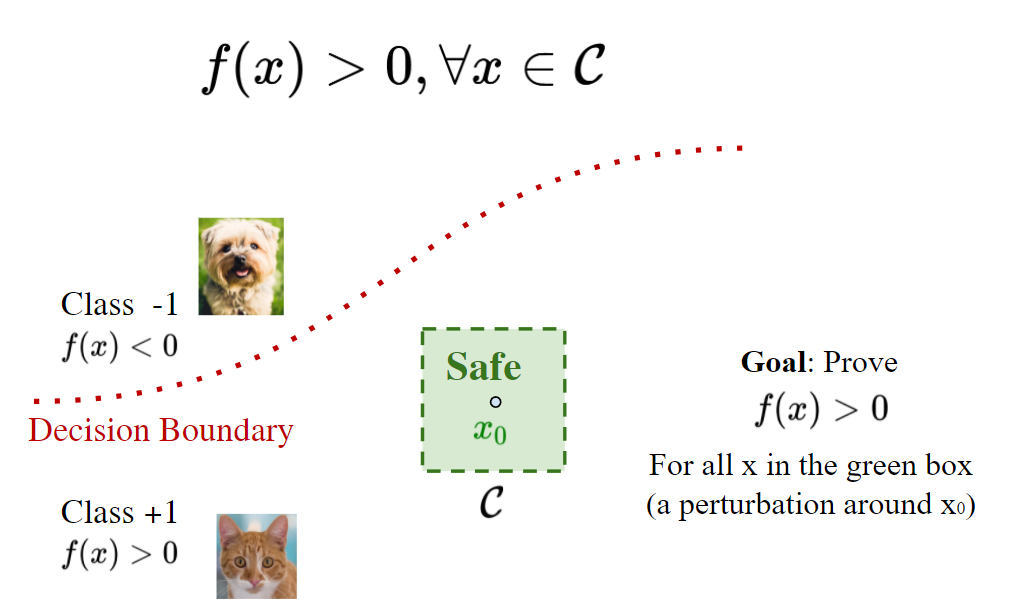# Part I - Introductionan short introduction to neural network verification.

Click here: Slides for Part I - Introduction (PDF)

tl;dr: In this part, we start with several concrete examples to demonstrate the importance of neural network verification. Then we present the formulations and fundamental mathematical problems solved by neural network verifiers. We also briefly introduce the detailed formulation of neural network verification, where existing verifiers can be divided into two categories: complete and incomplete verifiers. An overview of the evolving history of the neural network verifiers, along with significant performance improvements, will be presented, encouraging more efforts in this field.*****

A neural network verification tutorial presented by Huan Zhang, Kaidi Xu, Shiqi Wang and Cho-Jui Hsieh

☕ Pudhina theme by Knhash 🛠️